### D.13 The har­monic os­cil­la­tor and un­cer­tainty

The given qual­i­ta­tive ex­pla­na­tion of the ground state of the har­monic os­cil­la­tor in terms of the un­cer­tainty prin­ci­ple is ques­tion­able. In par­tic­u­lar, po­si­tion, lin­ear mo­men­tum, po­ten­tial en­ergy, and ki­netic en­ergy are un­cer­tain for the ground state. This note gives a solid ar­gu­ment, but it uses some ad­vanced ideas dis­cussed in chap­ter 4.4 and 4.5.3.

As ex­plained more fully in chap­ter 4.4, the ex­pec­ta­tion value of the ki­netic en­ergy is de­fined as the av­er­age value ex­pected for ki­netic en­ergy mea­sure­ments. Sim­i­larly, the ex­pec­ta­tion value of the po­ten­tial en­ergy is de­fined as the av­er­age value ex­pected for po­ten­tial en­ergy mea­sure­ments.

From the pre­cise form of ex­pec­ta­tion val­ues in quan­tum me­chan­ics, it fol­lows that to­tal en­ergy must be the sum of the ki­netic and po­ten­tial en­ergy ex­pec­ta­tion val­ues. For the har­monic os­cil­la­tor ground state, that gives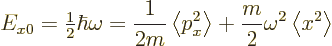Here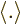stands for the av­er­age of the en­closed quan­tity. Only the mo­tion in the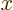-​di­rec­tion will be con­sid­ered here. The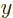and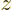di­rec­tions go ex­actly the same way.

Now any value of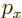can be writ­ten as equal to the av­er­age value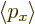plus a de­vi­a­tion from that av­er­age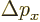. Then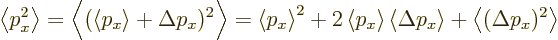Note that an av­er­age is a con­stant that is not af­fected by fur­ther av­er­ag­ing. Next note that the av­er­age ofis zero, oth­er­wise the av­er­age of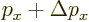would not be. So: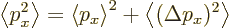Of course, a sim­i­lar ex­pres­sion holds for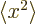, so the ground state en­ergy is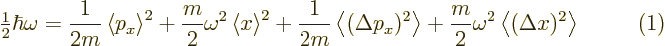Con­sider the last two terms. Call them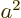and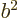for now. Note that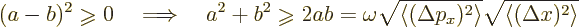as fol­lows from mul­ti­ply­ing out the square. The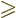be­comes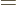when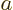and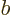are equal.

Now the first square root above is a mea­sure of the un­cer­tainty in. Ifis al­ways zero, thenis al­ways its av­er­age value, with­out any un­cer­tainty. Sim­i­larly, the sec­ond square root above is a mea­sure of the un­cer­tainty in. The Heisen­berg un­cer­tainty prin­ci­ple can be made quan­ti­ta­tive as, chap­ter 4.5.3,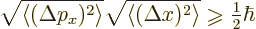There­fore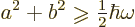So the min­i­mum value of the fi­nal two terms in the ex­pres­sion (1) for the ground state en­ergy is the com­plete ground state en­ergy. There­fore, in or­der that the right hand side in (1) does not ex­ceed the left hand side, the first two terms must be zero. So the av­er­age par­ti­cle mo­men­tum and po­si­tion are both zero. In ad­di­tion, for the es­ti­mates of the fi­nal two terms, equal­i­ties are needed, not in­equal­i­ties. That means thatmust be. That then means that the ex­pec­ta­tion ki­netic en­ergy must be the ex­pec­ta­tion po­ten­tial en­ergy. And the two must be the very min­i­mum al­lowed by the Heisen­berg re­la­tion; oth­er­wise there is still that in­equal­ity.